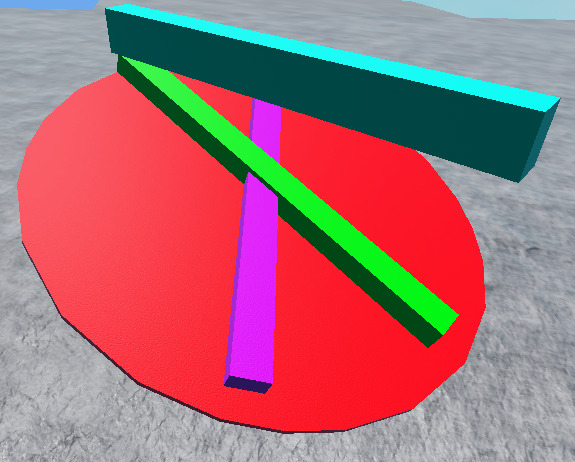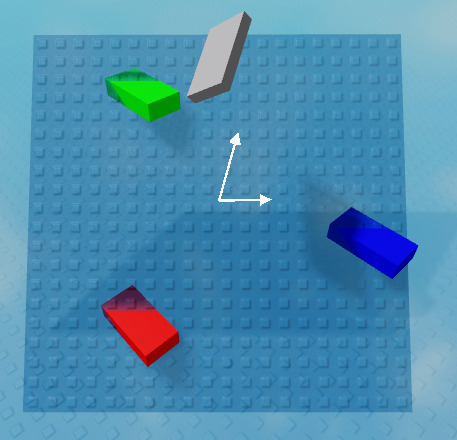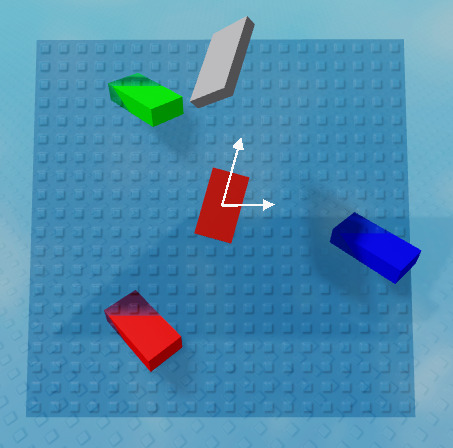# CFrame intersecting 3 positions

Hi
I want to construct a CFrame that intersects with 3 positions given. The positional part isn’t necessary, I just want the rotation that is needed to have the 3 parts inside the CFrame “plane”.

I want to use this to rotate a part using a BodyGyro.

1 Like

A CFrame describes a position and orientation in space. Something “intersecting” with a CFrame doesn’t make sense. A CFrame alone doesn’t describe a plane, for that you’ll need a point and a vector or three points. So e.g. the position of the CFrame and the LookVector. Is this what you mean? If not, please draw a figure or try and explain it in more detail.

2 Likes

I want a part to go through 3 positions.

The CFrame of that part has to be calculated from these three positions.

Basically an imaginary plane that contains the 3 positions, the physical part needs to be aligned on that plane.

To further clear up my question:

Let’s say I have these three parts which I only know the positions of:

I want to create a part that intersects these three parts right in the middle. The size of this part doesn’t matter. Something like this:

It should go exactly through the middle of each part, but this is the best I could approximate

I can’t give an example but

Finding the midpoint is easy. (pos1+pos2+pos3)/3

Set the size based on the distance between the pos, this might need some experimenting

Then you need to find the slope of each axis. You can run atan or some inverse trig func to get the angle, then set the part’s orientation as that.

1 Like
``````-- assuming a->b->c is CCW
local function PlaneCFrame(a, b, c)
local ab, ac = b - a, c - a
return CFrame.fromMatrix((a + b + c) / 3, ab, ab:Cross(ac))
end
``````

should work

edit: yup``````local plane = Instance.new("Part")
plane.Size = Vector3.new(30, 0.5, 30)
plane.Anchored = true
plane.CFrame = PlaneCFrame(workspace.a.Position, workspace.b.Position, workspace.c.Position)
plane.Parent = workspace
``````
1 Like

Thanks!
I’m still having some issues with that function however…
Sometimes the orientation of the plane “flips”, meaning that suddenly the plane is rotated the other way. it still intersects with the parts, but is rotated 180 degrees. I need to update this frequently, so it is important that the orientation stays the same. Is there a way to constrain it, so it always looks up?

This issue makes it behave rather unstable when updating every frame.

That will happen if a->b->c is clockwise (from the top down)

Easy fix is to flip the upvector if its negative

``````local function PlaneCFrame(a, b, c)
local ab, ac = b - a, c - a
local up = ab:Cross(ac)
if up.Y < 0 then up = -up end
return CFrame.fromMatrix((a + b + c) / 3, ab, up)
end
``````
1 Like

Thank you!
That works perfectly. I would have never come up with this.
Thanks again for your help and @myaltaccountsthis, @ThanksRoBama to.

Just a heads up that this doesn’t take into account cases where a->b->c are in a straight line or on top of each other, because there’s no solution. You’ll have to treat that as a special case and do something else:

``````-- assuming a->b->c is CCW
local EPS = 0.000001
local function PlaneCFrame(a, b, c)
local ab, ac = b - a, c - a
local up = ab:Cross(ac)

-- check if colinear
if up.X < EPS and up.Y < EPS and up.Z < EPS then
-- straight line
print("is straight")
end

if up.Y < 0 then up = -up end
return CFrame.fromMatrix((a + b + c) / 3, ab, up)
end
``````
1 Like

What needs to be done if they are colinear? Does there exist no solution?

Also is it necessary for a → b → c to be CCW? In my tests it doesn’t seem to matter…

EDIT: Is it possible for part to keep it’s orientation?
I want it to still be rotated along the plane, but it should look in the same direction.
An image to clear that up:The blue part is the part to be rotated, the green part is the desired result (still looking in the same direction). And the purple part is the result I’m sometimes getting (unwanted).

Anything’s possible!

Just to clarify, is this your new question?

Given a plane defined by three points, project a part onto that plane by:

• moving it’s position to the closest point on the plane, and
• rotating it the minimal amount needed to make its LookVector lie in the plane.
• Is the first bullet right or do you want to change the parts position to be the average of the three points?

• Do you also need the RightVector to lie in the plane? I.e. in that pic, if the blue part was twisted along its length by 45 degrees, what should the green part look like?

It might also help if you described what application you need this for1 Like

There’s no solution, because an infinite number of planes can be “rotated around” that line. The best option is probably to pick the normal that’s closest to the previous one in these cases. The simplest option is to just arbitrarily pick some other vector to define it.

It was, that’s what was causing your flipping issue. The requirement was fixed in the second version.

1 Like

I want to use this to make a part float on an ocean wave.

The wave height is calculated from three corner positions of the part. The calculated CFrame is used as input on a BodyGyro that tilts the part to rotate along the wave.

The part’s CFrame shouldn’t rotate along the y-axis, only along the (local) x and z-axes.

The positional part of the CFrame is not needed for my use-case, since the BodyGyro throws it away anyway.

1 Like

Seems like you might be better off adding a VectorForce to each corner of the part depending on how deep under the surface you are.

I understand what you mean, but its a bit hard to quantify this.

Like it seems like a simple problem at first—project the RightVector and LookVector onto the plane, and use the UpVector as the plane normal.

However, when you project the Right/Look vectors onto the plane, they won’t usually be 90 degrees apart anymore:My solution for now is just to decide to align the LookVector and set the RightVector accordingly:That seems to work fine for visuals at least. There’s probably some more sophistocated solution where you find the minimum rotation required to have the part lie in the plane… but that is beyond me at the moment.

Final code:

``````local part = workspace.Part
local a, b, c = workspace.A, workspace.B, workspace.C

local proj = part:Clone()
proj.BrickColor = BrickColor.Red()
proj.Parent = workspace

local plane = Instance.new("Part")
plane.Size = Vector3.new(20, 0.1, 20)
plane.Anchored = true
plane.Transparency = 0.5
plane.BrickColor = BrickColor.Blue()
plane.Parent = workspace

-- computes some CFrame on the plane formed by a, b, and c
-- note: only used to orient the blue plane
local function PlaneCFrame(a, b, c)
local ab, ac = b - a, c - a
local up = ab:Cross(ac)
if up.Y < 0 then up = -up end
return CFrame.fromMatrix((a + b + c) / 3, ab, up)
end

-- projects a point vertically onto the plane
-- vec: point to project
-- p: point on the plane
-- n: unit normal of plane
local function ProjectVertically(vec, p, n)
local off = vec - p
local y = -(n.X*off.X + n.Z*off.Z)/n.Y
return p + Vector3.new(off.X, y, off.Z)
end

-- projects cf vertically onto the plane formed by a, b, and c
-- such that cf.LookVector does not change rotation around
-- the y axis and that cf.RightVector lies in the plane
local function ProjectToPlane(cf, a, b, c)
local ab, bc = b - a, c - b
local n = ab:Cross(bc).Unit -- normal vector of plane

if n.Y < 0 then n = -n end -- points up

-- project lookVector to plane vertically:
local look = cf.LookVector
look = look - look:Dot(n)*n
local right = look:Cross(n)

-- optional: project position on plane straight down
local pos = ProjectVertically(cf.Position, a, n)

return CFrame.fromMatrix(pos, right, n, -look)
end

game:GetService("RunService").Stepped:Connect(function()
proj.CFrame = ProjectToPlane(part.CFrame, a.Position, b.Position, c.Position)
plane.CFrame = PlaneCFrame(a.Position, b.Position, c.Position)
end)
``````
2 Likes

That is perfect for me.